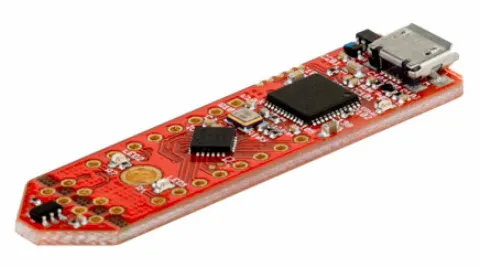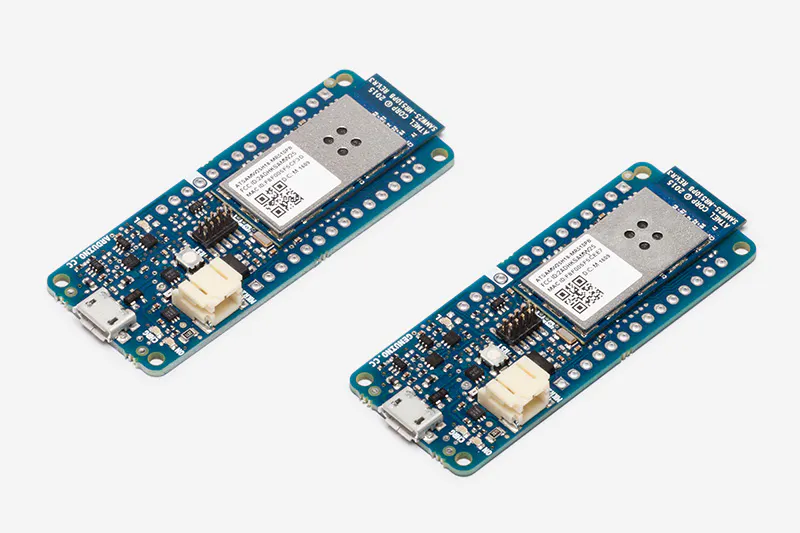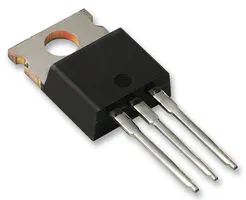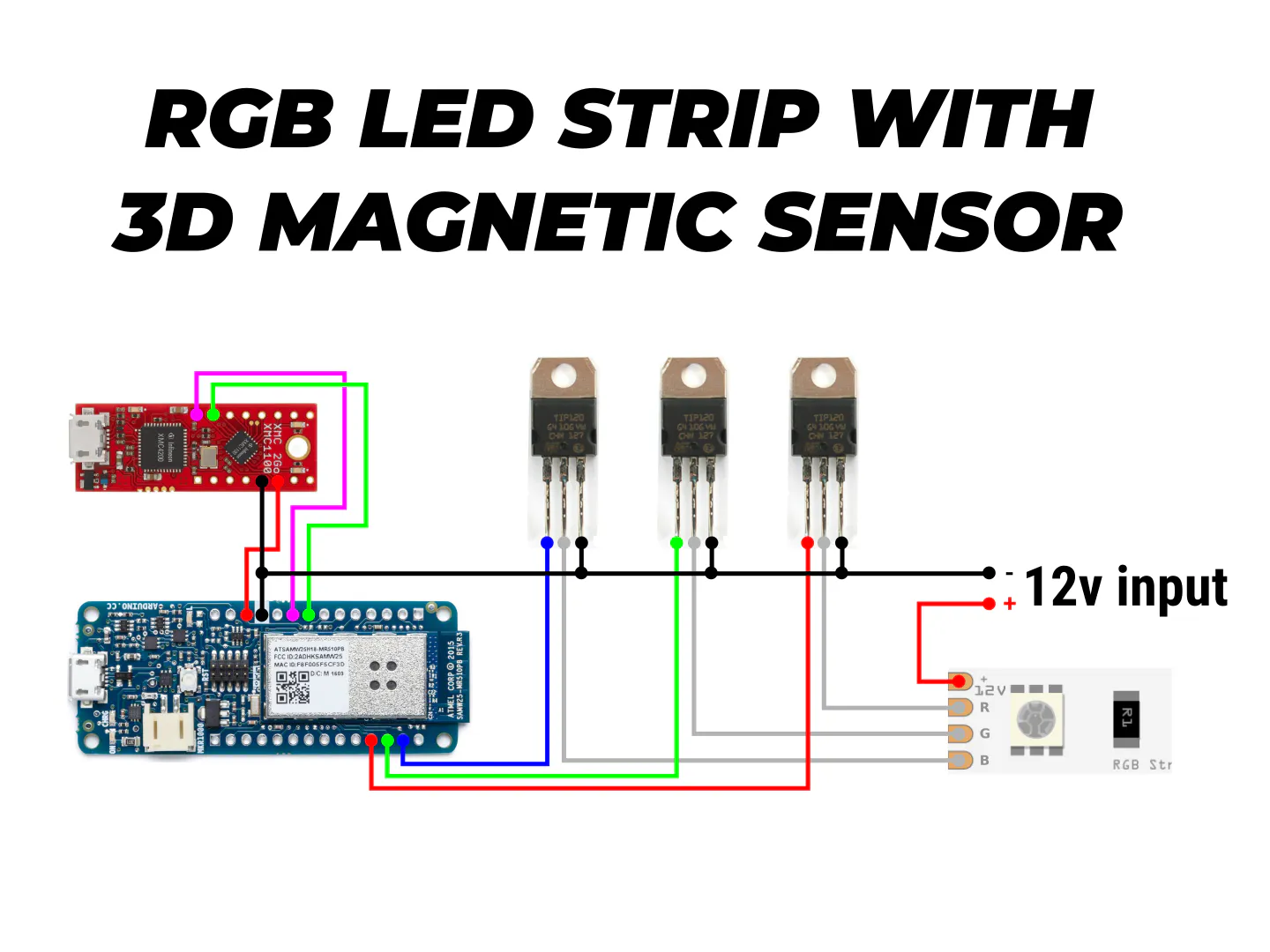# RGB Infinity Mirror with 3D Magnetic Sensor

An infinity mirror with an RGB LED strip that can change its color using Infineon's 3D magnetic sensor knob connected to an Arduino MKR1000.

IntermediateFull instructions provided3 hours3,676

## Things used in this project

### Hardware componentsInfineon 3D Magnetic Sensor 2Go
×1Arduino MKR1000
×1Darlington High Power Transistor
×3
 RGB LED Strip
×1Jumper wires (generic)
×1

### Software apps and online servicesArduino IDE

### Hand tools and fabrication machinesSoldering iron (generic)

## Schematics

### Connection Diagram

Upload the codes to the 3D Magnetic sensor kit and the MKR1000, and connect everything according to this diagram.## Code

### 3D Magnetic Sensor code

Arduino
Upload this to the 3D Magnetic sensor after installing the board in the Arduino IDE
```#include <Tle493d_w2b6.h>
const float pi = (22.0 / 7.0);
bool switchPressed;

Tle493d_w2b6 Tle493dMagnetic3DSensor = Tle493d_w2b6();
void setup() {
Serial.begin(9600);
Tle493dMagnetic3DSensor.begin();
Tle493dMagnetic3DSensor.begin();
}

void loop() {
Tle493dMagnetic3DSensor.updateData();
delay(100);
isSwitchPressed();

float angle = pi + Tle493dMagnetic3DSensor.getAzimuth();
angle = (angle * 180.0) / pi;

if (!switchPressed) {
Serial.println(angle);
} else if (switchPressed) {
//Do here whatever you want to do when the switch is pressed
}
}

void isSwitchPressed() {
if (Tle493dMagnetic3DSensor.getNorm() < 55) {
switchPressed = false;
}
else if (Tle493dMagnetic3DSensor.getNorm() > 85) {
switchPressed = true;
}
}
```

### Arduino MKR1000 code

Arduino
Upload this code to the Arduino MKR1000, and connect everything according to the connection diagram (after uploading the code to the XMC2Go)
```float deg;
int pinRed = 2;
int pinGreen = 3;
int pinBlue = 4;

void setup() {
Serial.begin(9600);
Serial1.begin(9600);

pinMode(pinRed, OUTPUT);
pinMode(pinGreen, OUTPUT);
pinMode(pinBlue, OUTPUT);
}

void loop() {
if (Serial1.available() > 0) {
deg = Serial1.parseFloat();
setLedColorHSV(deg, 1, 1);
}
}

void setLedColorHSV(int h, double s, double v) {
//this is the algorithm to convert from RGB to HSV
double r = 0;
double g = 0;
double b = 0;

double hf = h / 60.0;

int i = (int)floor(h / 60.0);
double f = h / 60.0 - i;
double pv = v * (1 - s);
double qv = v * (1 - s * f);
double tv = v * (1 - s * (1 - f));

switch (i) {
case 0:
r = v;
g = tv;
b = pv;
break;
case 1:
r = qv;
g = v;
b = pv;
break;
case 2:
r = pv;
g = v;
b = tv;
break;
case 3:
r = pv;
g = qv;
b = v;
break;
case 4:
r = tv;
g = pv;
b = v;
break;
case 5:
r = v;
g = pv;
b = qv;
break;
}

//set each component to a integer value between 0 and 255
int red = constrain((int)255 * r, 0, 255);
int green = constrain((int)255 * g, 0, 255);
int blue = constrain((int)255 * b, 0, 255);

Serial.print("Red: ");    Serial.print(red);
Serial.print(" Green: "); Serial.print(green);
Serial.print(" Blue: ");   Serial.print(blue);
Serial.println();

analogWrite(pinRed, red);
analogWrite(pinGreen, green);
analogWrite(pinBlue, blue);
}
```